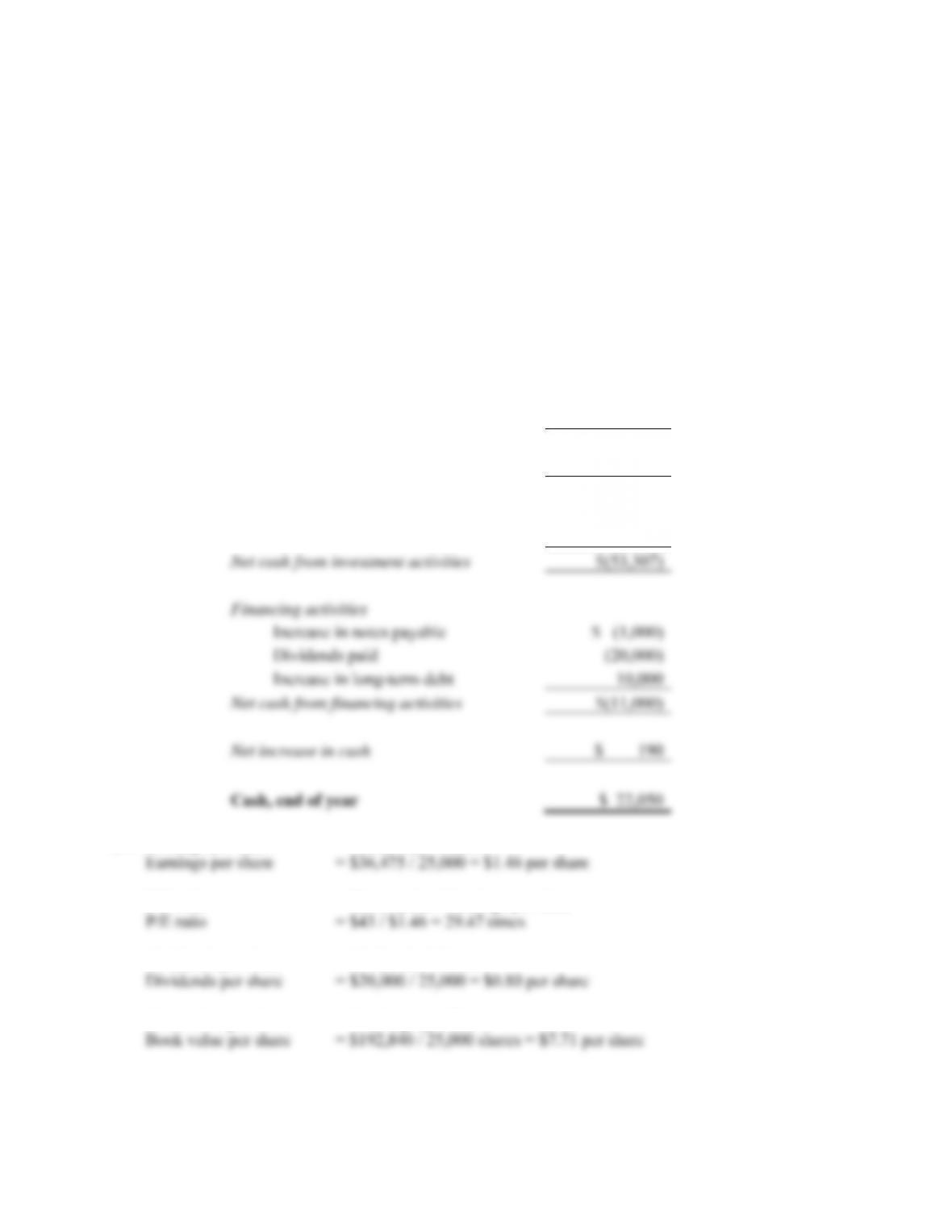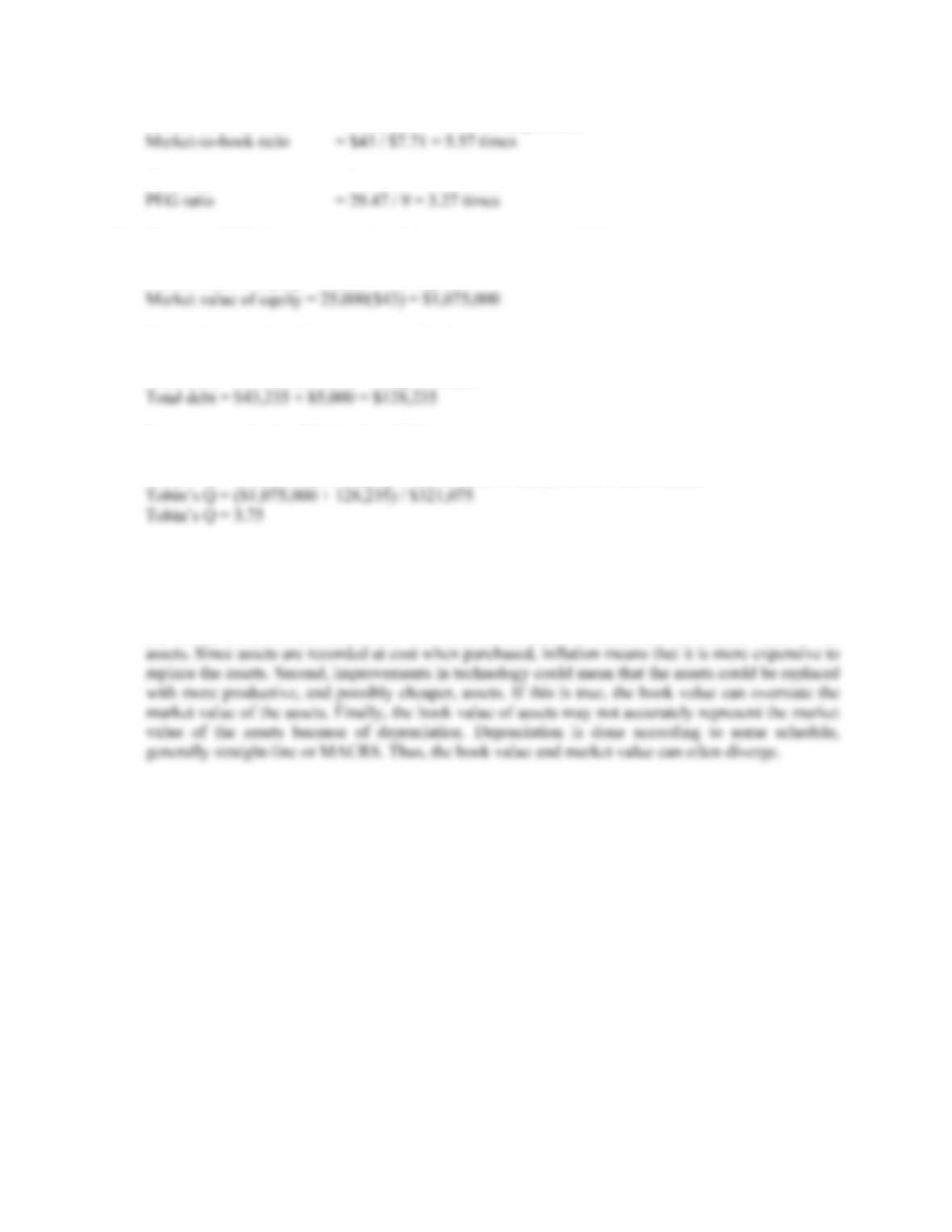Type
Quiz
Book Title
Fundamentals of Corporate Finance Standard Edition 9th Edition
ISBN 13
978-0073382395

### 978-0073382395 Chapter 3 Questions and Problems 28-30

April 3, 2019CHAPTER 3 B-27
28. SMOLIRA GOLF CORP.
Statement of Cash Flows
For 2009
Cash, beginning of the year \$ 21,860
Operating activities
Net income \$ 36,475
Plus:
Depreciation \$ 26,850
Increase in accounts payable 3,530
Increase in other current liabilities 1,742
Less:
Increase in accounts receivable \$ (2,534)
Increase in inventory (1,566)
Net cash from operating activities \$ 64,497
Investment activities
Fixed asset acquisition \$(53,307)
29. Earnings per share = Net income / Shares
P/E ratio = Shares price / Earnings per share
Dividends per share = Dividends / Shares
Book value per share = Total equity / SharesB-28 SOLUTIONS
Market-to-book ratio = Share price / Book value per share
PEG ratio = P/E ratio / Growth rate
30. First, we will find the market value of the company’s equity, which is:
Market value of equity = Shares × Share price
The total book value of the company’s debt is:
Total debt = Current liabilities + Long-term debt
Now we can calculate Tobin’s Q, which is:
Tobin’s Q = (Market value of equity + Book value of debt) / Book value of assets
Using the book value of debt implicitly assumes that the book value of debt is equal to the market
value of debt. This will be discussed in more detail in later chapters, but this assumption is generally
true. Using the book value of assets assumes that the assets can be replaced at the current value on
the balance sheet. There are several reasons this assumption could be flawed. First, inflation during
the life of the assets can cause the book value of the assets to understate the market value of the